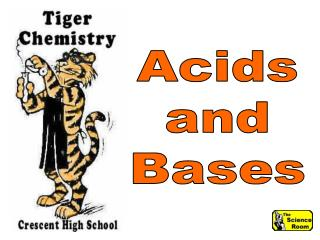DownloadDownload PresentationAcids and Bases

# Acids and Bases

Télécharger la présentation## Acids and Bases

- - - - - - - - - - - - - - - - - - - - - - - - - - - E N D - - - - - - - - - - - - - - - - - - - - - - - - - - -
##### Presentation Transcript

1. Acids and Bases

2. Acids & Bases

3. Water and acid combine in an exothermic reaction - releasing large amounts of heat.

4. Water and acid combine in an exothermic reaction - releasing large amounts of heat.

5. Acid - Base Theories • Lavoisier • Arrhenius • Bronsted-Lowry • Lewis

6. AntoineLavoisier

7. Lavoisier - 1777 Acidity is caused by the presence of oxygen in the compound.

8. Lavoisier - 1777 He even created the word oxygen - from the Greek oxys, "sour" and genes, "born"

9. Lavoisier - 1777 Oxygen means "acid maker".

10. Lavoisier - 1777 Lavoisier's idea about acids turned out to be wrong...

11. Lavoisier - 1777 but it is historically important since it is the first scientific attempt to chemically characterize acids and bases.

12. Each theory includes more substances as acids and bases Arrhenius Bronsted-Lowry Lewis

13. SvanteAugustArrhenius

14. Arrhenius Acid Any substance that produces hydrogen ions, H+, in water solution.

15. Arrhenius Base Any substance that produces hydroxide ions, OH-, in water solution.

16. In a formula, such as HCl, the positive part of a Arrhenius acid will ALWAYS be hydrogen.

17. In a formula, such as NaOH, the negative part of a Arrhenius base will ALWAYS be hydroxide.

18. Arrhenius acids and bases, and soluble salts, are called electrolytes.

19. When each dissolves, ions are released that conduct electricity.

20. If a solution has NO ions, it cannot conduct electricity.

21. Bronsted-Lowry Theory JohannesNicolausBronsted

22. Bronsted-Lowry Theory ThomasMartinLowry

23. Bronsted-Lowry Acid Any substance that acts as a proton donor.

24. Bronsted-Lowry Acid A B-L acid MUST have hydrogen somewhere in its formula.

25. Bronsted-Lowry Acid When an electron is removed from a hydrogen atom... - +

26. Bronsted-Lowry Acid a hydrogen ion is produced - a proton. +

27. Bronsted-Lowry Base Any substance that acts as a proton acceptor.

28. Bronsted-Lowry Base Most negative ions can acts as B-L bases.

29. Bronsted-Lowry Theory The acid/base definitions are broadened because no specific ion must be formed, although hydrogen is the only source of protons.

30. What is the formula for the hydronium ion?

31. H3O+

32. Bronsted-Lowry Theory The hydronium ion is formed by combining a hydrogen ion and a water molecule. H2O + H+ H3O+

33. Bronsted-Lowry Theory conjugate base

34. Bronsted-Lowry Theory A conjugate base is the particle that remains AFTER an acid gives up a proton.

35. Bronsted-Lowry Theory conjugate acid

36. Bronsted-Lowry Theory A conjugate acid is the particle formed when a base accepts a proton.

37. HCl + H2O Cl- + H3O+ Identify the B-L acid in this equation. What gives up a proton from the left side to the right?

38. HCl + H2O Cl- + H3O+ HCl donates a proton, it is the B-L acid.

39. HCl + H2O Cl- + H3O+ Identify the B-L base in this equation. What gains a proton from the left side to the right?

40. HCl + H2O Cl- + H3O+ H2O accepts a proton, it is the B-L base.

41. HCl + H2O Cl- + H3O+ Identify the conjugate base in this equation. What is left when the acid gives up a proton?

42. HCl + H2O Cl- + H3O+ Cl- is left when HCl gives up a proton, it is the conjugate base.

43. HCl + H2O Cl- + H3O+ Identify the conjugate acid in this equation. What is formed when the base accepts a proton?

44. HCl + H2O Cl- + H3O+ H3O+ is formed when water accepts a proton, it is the conjugate acid.

45. HCl + H2O Cl- + H3O+ acids have conjugate bases

46. HCl + H2OCl- +H3O+ bases have conjugate acids

47. Remove a proton from an acid to get its conjugate base. H2SO4 HI H2O HSO4-

48. Remove a proton from an acid to get its conjugate base. H2SO4 HI H2O HSO4- I-

49. Remove a proton from an acid to get its conjugate base. H2SO4 HI H2O HSO4- I- OH-